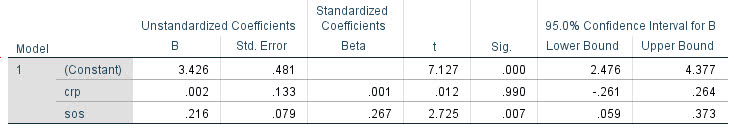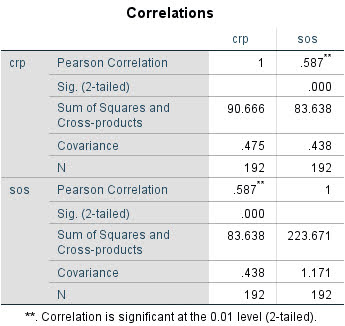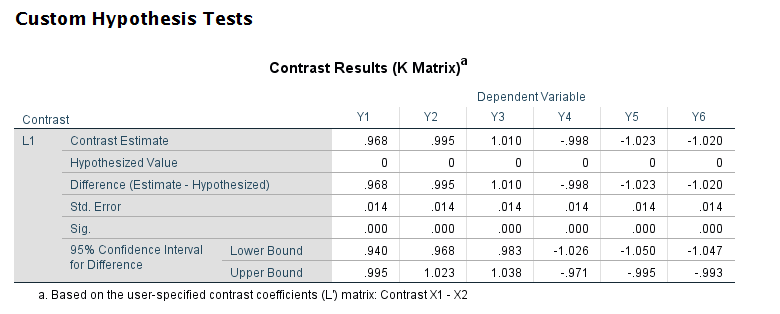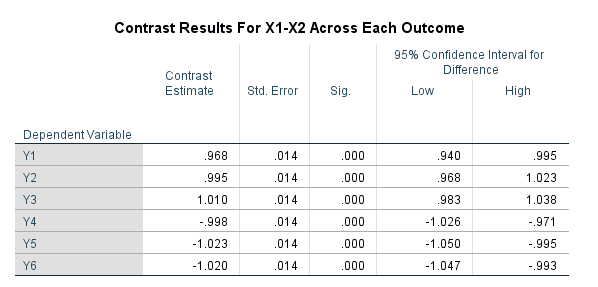# Difference in independent effects for multivariate analysis (SPSS)

For some reason my various posts on testing differences in coefficients are fairly high in google search results. Arden Roeder writes in with another related question on this:

Good evening Dr. Wheeler,

I am a PhD candidate at the University of Oklahoma working on the final phases of data analysis for my dissertation. I found an article on your website that almost answers a question I have about a potential statistical test, and I’m hoping you might be able to help me fill in the gaps.

If you are willing to help me out, I would greatly appreciate it, and if not, I completely understand!

Here’s the setup: I have two independent variables (one measured on a 6-point Likert scale, the other on a 7-point) and six dependent variables. I have hypothesized that IV1 would be a stronger predictor of three of the DVs, and that IV2 would be a stronger predictor of the other three DVs. I ran multiple linear regression tests for each of the DVs, so I have the outputs for those. I know I can compare the standardized betas just to see strength, but what I’d like to know is how I can determine whether the difference between the beta weights is significant, and then to assess this for each of the six DVs.

From reading through your post, it seems like the fourth scenario you set up is quite close to what I’m trying to do, but I’m not sure how to translate the covariance output I have (I’m using SPSS) to what you’ve displayed here. Can I simply square the standard errors I have to get the numbers on the diagonal, and then grab the covariance from the SPSS output and solve accordingly? (I also reviewed your writing here about using the GLM procedure as well, but can’t seem to align my outputs with your examples there either.)

Here’s a sample of the numbers I’m working with:Any insights you can offer on 1) whether this is the right test to give me the answers I’m looking for about whether the betas are significantly different and 2) how to set up and interpret the results correctly would be a tremendous help.

For 1, yes I think this is an appropriate way to set up the problem. For 2, if sticking to SPSS it is fairly simple syntax in GLM:

``````*****************************.
*Contrasts with X1 - X2 effect across the variables.
GLM Y1 Y2 Y3 Y4 Y5 Y6 WITH X1 X2
/DESIGN=X1 X2
/PRINT PARAMETER
/LMATRIX = "T1"
X1  1
X2 -1.
*****************************.``````

To get this to do the standardized coefficients, Z-score your variables before the GLM command (this is assuming you are estimating a linear model, and not a non-linear model like logit/Poisson). (I have full simulation SPSS code at the end of the post illustrating.)

Note that when I say the covariance between beta coefficients, this is NOT the same thing as the covariance between the original metrics. So the correlation matrix for X1 vs X2 here does not give us the information we need.

For 2, the reporting part, you can see the Contrast results K matrix table in SPSS. I would just transpose that table, make a footnote/title this is testing X1 – X2, and then just keep the columns you want. So here is the original SPSS contrast table for this result:And here is how I would clean up the table and report the tests:# SPSS Simulation Code

Here I just simulate independent X’s, and give the Y’s consistent effects. You could also simulate multivariate data with specified correlations if you wanted to though.

``````****************************************************.
* Simulated data to illustrate coefficient diff.
* tests.
SET SEED 10.
INPUT PROGRAM.
LOOP #i = 1 TO 10000.
COMPUTE Id = #i.
END CASE.
END LOOP.
END FILE.
END INPUT PROGRAM.

COMPUTE X1 = RV.NORMAL(0,1).
COMPUTE X2 = RV.NORMAL(0,1).
COMPUTE Y1 = 1.5*X1 + 0.5*X2 + RV.NORMAL(0,1).
COMPUTE Y2 = 1.5*X1 + 0.5*X2 + RV.NORMAL(0,1).
COMPUTE Y3 = 1.5*X1 + 0.5*X2 + RV.NORMAL(0,1).
COMPUTE Y4 = 0.5*X1 + 1.5*X2 + RV.NORMAL(0,1).
COMPUTE Y5 = 0.5*X1 + 1.5*X2 + RV.NORMAL(0,1).
COMPUTE Y6 = 0.5*X1 + 1.5*X2 + RV.NORMAL(0,1).
EXECUTE.

*Contrasts with X1 - X2 effect across the variables.
GLM Y1 Y2 Y3 Y4 Y5 Y6 WITH X1 X2
/DESIGN=X1 X2
/PRINT PARAMETER
/LMATRIX = "Contrast X1 - X2"
X1  1
X2 -1.

*And here is an example stacking equations and using EMMEANS.
*Stacking equation approach, can work for various.
*Generalized linear models, etc.
VARSTOCASES
/MAKE Y FROM Y1 TO Y6
/Index Outcome.

GENLIN Y BY Outcome WITH X1 X2
/MODEL Outcome X1 X2 Outcome*X1 Outcome*X2
/CRITERIA COVB=ROBUST
/REPEATED SUBJECT=Id CORRTYPE=UNSTRUCTURED
/EMMEANS TABLES=Outcome CONTROL= X1(1) X2(-1).
****************************************************.``````

# Some more testing coefficient contrasts: Multinomial models and indirect effects

Testing the equality of two coefficients is one of my more popular posts. This is a good thing — often more interesting hypotheses are to test two parameters against each other, as opposed to a strict null hypothesis of a coefficient against zero. Every now an then I get questions about applying this idea to new situations in which it is not always straightforward how to figure out. So here are a few examples using demonstration R code.

# Multinomial Models

One question I received about applying the advice was to test coefficients across different contrasts in multinomial models. It may not seem obvious, but the general approach of extracting out the coefficients and the covariance between those estimates works the same way as most regression equations.

So in a quick example in R:

``````library(nnet)
data(mtcars)
library(car)

mtcars\$cyl <- as.factor(mtcars\$cyl)
mtcars\$am <- as.factor(mtcars\$am)
mod <- multinom(cyl ~ am + hp, data=mtcars, Hess=TRUE)
summary(mod)``````

And the estimates for `mod` are:

``````> summary(mod)
Call:
multinom(formula = cyl ~ am + hp, data = mtcars)

Coefficients:
(Intercept)       am1        hp
6   -42.03847  -3.77398 0.4147498
8   -92.30944 -26.27554 0.7836576

Std. Errors:
(Intercept)       am1        hp
6    27.77917  3.256003 0.2747842
8    31.93525 46.854100 0.2559052

Residual Deviance: 7.702737
AIC: 19.70274 ``````

So say we want to test whether the `hp` effect is the same for `6` cylinders vs `8` cylinders. To test that, we just grab the covariance and construct our test:

``````#Example constructing test by hand
v <- vcov(mod)
c <- coef(mod)
dif <- c[1,3] - c[2,3]
se <- sqrt( v[3,3] + v[6,6] - 2*v[3,6])
z <- dif/se
#test stat, standard error, and two-tailed p-value
dif;se;2*(1 - pnorm(abs(z)))``````

Which we end up with a p-value of `0.0002505233`, so we would reject the null that these two effects are equal to one another. Note to get the variance-covariance estimates for the parameters you need to set `Hess=TRUE` in the `multinom` call.

Another easier way though is to use the `car` libraries function `linearHypothesis` to conduct the same test:

``````> linearHypothesis(mod,c("6:hp = 8:hp"),test="Chisq")
Linear hypothesis test

Hypothesis:
6:hp - 8:hp = 0

Model 1: restricted model
Model 2: cyl ~ am + hp

Df  Chisq Pr(>Chisq)
1
2  1 13.408  0.0002505 ***
---
Signif. codes:  0 ‘***’ 0.001 ‘**’ 0.01 ‘*’ 0.05 ‘.’ 0.1 ‘ ’ 1``````

You can see although this is in terms of a Chi-square test, it results in the same p-value. The Wald test however can be extended to testing multiple coefficient equalities, and a popular one for multinomial models is to test if any coefficients change across different levels of the dependent categories. The idea behind that test is to see if you can collapse that category with another that is equivalent.

To do that test, I created a function that does all of the contrasts at once:

``````#Creating function to return tests for all coefficient equalities at once
all_tests <- function(model){
v <- colnames(coef(model))
d <- rownames(coef(model))
allpairs <- combn(d,2,simplify=FALSE)
totn <- length(allpairs) + length(d)
results <- data.frame(ord=1:totn)
results\$contrast <- ""
results\$test <- ""
results\$Df <- NULL
results\$Chisq <- NULL
results\$pvalue <- NULL
iter <- 0
for (i in allpairs){
iter <- iter + 1
l <- paste0(i,":",v)
r <- paste0(i,":",v)
test <- paste0(l," = ",r)
temp_res <- linearHypothesis(model,test,test="Chisq")
results\$contrast[iter] <- paste0(i," vs ",i)
results\$test[iter] <- paste(test,collapse=" and ")
results\$Df[iter] <- temp_res\$Df
results\$Chisq[iter] <- temp_res\$Chisq
results\$pvalue[iter] <- temp_res\$Pr
}
ref <- model\$lab[!(model\$lab %in% d)]
for (i in d){
iter <- iter + 1
test <- paste0(i,":",v," = 0")
temp_res <- linearHypothesis(model,test,test="Chisq")
results\$contrast[iter] <- paste0(i," vs ",ref)
results\$test[iter] <- paste(test,collapse=" and ")
results\$Df[iter] <- temp_res\$Df
results\$Chisq[iter] <- temp_res\$Chisq
results\$pvalue[iter] <- temp_res\$Pr
}
return(results)
}``````

Not only does this construct the test of the observed categories, but also tests whether each set of coefficients is simultaneously zero, which is the appropriate contrast for the referent category.

``````> all_tests(mod)
ord contrast                                                            test Df        Chisq       pvalue
1   1   6 vs 8 6:(Intercept) = 8:(Intercept) and 6:am1 = 8:am1 and 6:hp = 8:hp  3    17.533511 0.0005488491
2   2   6 vs 4                    6:(Intercept) = 0 and 6:am1 = 0 and 6:hp = 0  3     5.941417 0.1144954481
3   3   8 vs 4                    8:(Intercept) = 0 and 8:am1 = 0 and 8:hp = 0  3 44080.662112 0.0000000000``````

User beware of multiple testing with this, as I am not sure as to the appropriate post-hoc correction here when examining so many hypotheses. This example with just three is obviously not a big deal, but with more categories you get n choose 2, or `(n*(n-1))/2` total contrasts.

# Testing the equality of multiple indirect effects

Another example I was asked about recently was testing whether you could use the same procedure to calculate indirect effects (popular in moderation and mediation analysis). Those end up being a bit more tricky, as to define the variance and covariance between those indirect effects we are not just dealing with adding and subtracting values of the original parameters, but are considering multiplications.

Thus to estimate the standard error and covariance parameters of indirect effects folks often use the delta method. In R using the `lavaan` library, here is an example (just taken from a code snippet Yves Rosseel posted himself), to estimate the variance-covariance matrix model defined indirect parameters.

``````#function taken from post in
library(lavaan)
vcov.def <- function(model){
m <- model
orig <- vcov(m)
free <- m@Fit@x
jac <- lavaan:::lavJacobianD(func = m@Model@def.function, x = free)
vcov_def <- jac %*% orig %*% t(jac)
estNames <- subset(parameterEstimates(m),op==":=")
row.names(vcov_def) <- estNames\$lhs
colnames(vcov_def) <- estNames\$lhs
#I want to print the covariance table estimates to make sure the
#labels are in the correct order
estNames\$se2 <- sqrt(diag(vcov_def))
estNames\$difSE <- estNames\$se - estNames\$se2
print(estNames[,c('lhs','se','se2','difSE')])
print('If difSE is not zero, labels are not in right order')
return(vcov_def)
}``````

Now here is an example of testing individual parameter estimates for indirect effects.

``````set.seed(10)
n <- 100
X1 <- rnorm(n)
X2 <- rnorm(n)
X3 <- rnorm(n)
M <- 0.5*X1 + 0.4*X2 + 0.3*X3 + rnorm(n)
Y <- 0.1*X1 + 0.2*X2 + 0.3*X3 + 0.7*M + rnorm(n)
Data <- data.frame(X1 = X1, X2 = X2, X3 = X3, Y = Y, M = M)
model <- ' # direct effect
Y ~ X1 + X2 + X3 + d*M
# mediator
M ~ a*X1 + b*X2 + c*X3
# indirect effects
bd := b*d
cd := c*d
'
model_SP.fit <- sem(model, data = Data)
summary(model_SP.fit)

#now apply to your own sem model
defCov <- vcov.def(model_SP.fit)``````

Unfortunately as far as I know, the `linearHypothesis` function does not work for `lavaan` objects, so if we want to test whether the indirect effect of whether `ad = bd` we need to construct it by hand. But with the `vcov.def` function we have those covariance terms we needed.

``````#testing hypothesis that "ad = bd"
#so doing "ad - bd = 0"
model_SP.param <- parameterEstimates(model_SP.fit)
model_SP.defined <- subset(model_SP.param, op==":=")
dif <- model_SP.defined\$est - model_SP.defined\$est
var_dif <- defCov[1,1] + defCov[2,2] - 2*defCov[1,2]
#so the test standard error of the difference is
se_dif <- sqrt(var_dif)
#and the test statistic is
tstat <- dif/se_dif
#two tailed p-value
dif;se_dif;2*(1 - pnorm(abs(tstat)))``````

To test whether all three indirect parameters are equal to each other at once, one way is to estimate a restricted model, and then use a likelihood ratio test of the restricted vs the full model. It is pretty easy in `lavaan` to create coefficient restrictions, just set what was varying to only be one parameter:

``````restrict_model <- ' # direct effect
Y ~ X1 + X2 + X3 + d*M
# mediator
M ~ a*X1 + a*X2 + a*X3
# indirect effects